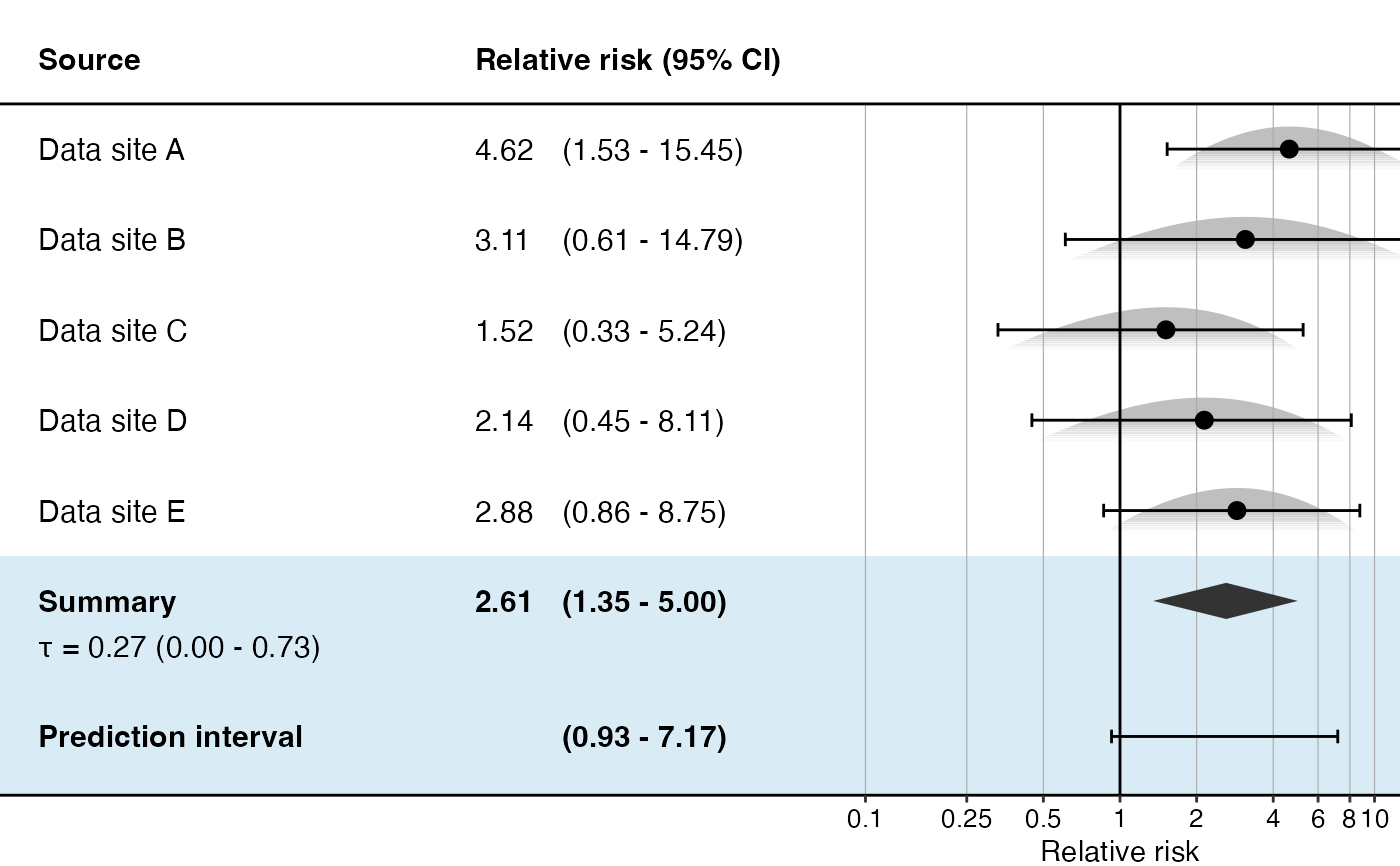Creates a forest plot of effect size estimates, including the summary estimate.

plotMetaAnalysisForest(
data,
labels,
estimate,
xLabel = "Relative risk",
summaryLabel = "Summary",
limits = c(0.1, 10),
alpha = 0.05,
showLikelihood = TRUE,
fileName = NULL
)

## Arguments

data

A data frame containing either normal, skew-normal, custom parametric, or grid likelihood data. One row per database.

labels

A vector of labels for the data sources.

estimate

The meta-analytic estimate as created using either ['computeFixedEffectMetaAnalysis()] or [computeBayesianMetaAnalysis()] function.

xLabel

The label on the x-axis: the name of the effect estimate.

summaryLabel

The label for the meta-analytic estimate.

limits

The limits of the effect size axis.

alpha

The alpha (expected type I error).

showLikelihood

Show the likelihood curve for each estimate?

fileName

Name of the file where the plot should be saved, for example 'plot.png'. See the function ggplot2::ggsave ifor supported file formats.

## Value

A Ggplot object. Use the ggplot2::ggsave function to save to file.

## Details

Creates a forest plot of effect size estimates, including a meta-analysis estimate.

## Examples

# Simulate some data for this example:
populations <- simulatePopulations()
labels <- paste("Data site", LETTERS[1:length(populations)])

# Fit a Cox regression at each data site, and approximate likelihood function:
fitModelInDatabase <- function(population) {
cyclopsData <- Cyclops::createCyclopsData(Surv(time, y) ~ x + strata(stratumId),
data = population,
modelType = "cox"
)
cyclopsFit <- Cyclops::fitCyclopsModel(cyclopsData)
approximation <- approximateLikelihood(cyclopsFit, parameter = "x", approximation = "custom")
return(approximation)
}
approximations <- lapply(populations, fitModelInDatabase)
approximations <- do.call("rbind", approximations)

# At study coordinating center, perform meta-analysis using per-site approximations:
estimate <- computeBayesianMetaAnalysis(approximations)
#> Detected data following custom parameric distribution
#> Performing MCMC. This may take a while
plotMetaAnalysisForest(approximations, labels, estimate)# (Estimates in this example will vary due to the random simulation)

`